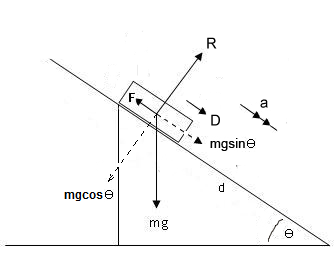# An object with a mass of 12 kg is on a plane with an incline of  -(3 pi)/8 . If it takes  4 N to start pushing the object down the plane and 2 N to keep pushing it, what are the coefficients of static and kinetic friction?

An object with a mass of 12 kg is on a plane with an incline of  -(3 pi)/8 . If it takes  4 N to start pushing the object down the plane and 2 N to keep pushing it, what are the coefficients of static and kinetic friction?

ReportAnswer 1

The static coefficient of friction is $2.5031$ (4dp)
The kinetic coefficient of friction is $2.4587$ (4dp)

#### Explanation:For our diagram, $m = 12 k g$, $\theta = \frac{3 \pi}{8}$

If we apply Newton's Second Law up perpendicular to the plane we get:

$R - m g \cos \theta = 0$
$\therefore R = 12 g \cos \left(\frac{3 \pi}{8}\right) \setminus \setminus N$

Initially it takes $4 N$ to start the object moving, so $D = 4$. If we Apply Newton's Second Law down parallel to the plane we get:

$D + m g \sin \theta - F = 0$
$\therefore F = 4 + 12 g \sin \left(\frac{3 \pi}{8}\right) \setminus \setminus N$

And the friction is related to the Reaction (Normal) Force by

$F = \mu R \implies 4 + 12 g \sin \left(\frac{3 \pi}{8}\right) = \mu \left(12 g \cos \left(\frac{3 \pi}{8}\right)\right)$
$\therefore \mu = \frac{4 + 12 g \sin \left(\frac{3 \pi}{8}\right)}{12 g \cos \left(\frac{3 \pi}{8}\right)}$
$\therefore \mu = 2.5030953 \ldots$

Once the object is moving the driving force is reduced from $4 N$ to $2 N$. Now $D = 3$, reapply Newton's Second Law down parallel to the plane and we get:

$D + m g \sin \theta - F = 0$
$\therefore F = 2 + 12 g \sin \left(\frac{3 \pi}{8}\right) \setminus \setminus N$

And the friction is related to the Reaction (Normal) Force by

 F = mu R => 2+12gsin ((3pi)/8) = mu (12gcos((3pi)/8) 
$\therefore \mu = \frac{2 + 12 g \sin \left(\frac{3 \pi}{8}\right)}{12 g \cos \left(\frac{3 \pi}{8}\right)}$
$\therefore \mu = 2.4586544 \ldots$

So the static coefficient of friction is $2.5031$ (4dp)
the kinetic coefficient of friction is $2.4587$ (4dp)

##### Add Answer of: An object with a mass of 12 kg is on a plane with an incline of  -(3 pi)/8 . If it takes  4 N to start pushing the object down the plane and 2 N to keep pushing it, what are the coefficients of static and kinetic friction?
Similar Homework Help Questions